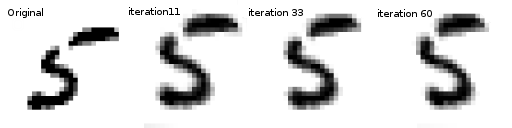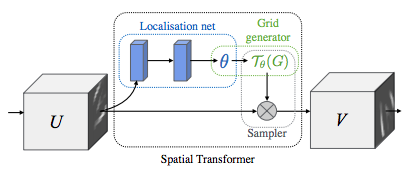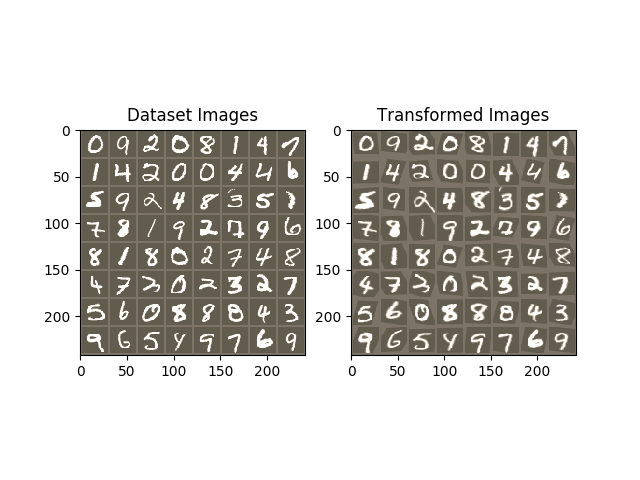# Spatial Transformer Networks TutorialIn this tutorial, you will learn how to augment your network using a visual attention mechanism called spatial transformer networks. You can read more about the spatial transformer networks in the DeepMind paper

Spatial transformer networks are a generalization of differentiable attention to any spatial transformation. Spatial transformer networks (STN for short) allow a neural network to learn how to perform spatial transformations on the input image in order to enhance the geometric invariance of the model. For example, it can crop a region of interest, scale and correct the orientation of an image. It can be a useful mechanism because CNNs are not invariant to rotation and scale and more general affine transformations.

One of the best things about STN is the ability to simply plug it into any existing CNN with very little modification.

```# License: BSD
# Author: Ghassen Hamrouni

from __future__ import print_function
import torch
import torch.nn as nn
import torch.nn.functional as F
import torch.optim as optim
import torchvision
from torchvision import datasets, transforms
import matplotlib.pyplot as plt
import numpy as np

plt.ion()   # interactive mode
```

In this post we experiment with the classic MNIST dataset. Using a standard convolutional network augmented with a spatial transformer network.

```use_cuda = torch.cuda.is_available()

# Training dataset
transform=transforms.Compose([
transforms.ToTensor(),
transforms.Normalize((0.1307,), (0.3081,))
])), batch_size=64, shuffle=True, num_workers=4)
# Test dataset
datasets.MNIST(root='.', train=False, transform=transforms.Compose([
transforms.ToTensor(),
transforms.Normalize((0.1307,), (0.3081,))
])), batch_size=64, shuffle=True, num_workers=4)
```

### Depicting spatial transformer networks

Spatial transformer networks boils down to three main components :

• The localization network is a regular CNN which regresses the transformation parameters. The transformation is never learned explicitly from this dataset, instead the network learns automatically the spatial transformations that enhances the global accuracy.
• The grid generator generates a grid of coordinates in the input image corresponding to each pixel from the output image.
• The sampler uses the parameters of the transformation and applies it to the input image.Note

We need the latest version of PyTorch that contains affine_grid and grid_sample modules.

```class Net(nn.Module):
def __init__(self):
super(Net, self).__init__()
self.conv1 = nn.Conv2d(1, 10, kernel_size=5)
self.conv2 = nn.Conv2d(10, 20, kernel_size=5)
self.conv2_drop = nn.Dropout2d()
self.fc1 = nn.Linear(320, 50)
self.fc2 = nn.Linear(50, 10)

# Spatial transformer localization-network
self.localization = nn.Sequential(
nn.Conv2d(1, 8, kernel_size=7),
nn.MaxPool2d(2, stride=2),
nn.ReLU(True),
nn.Conv2d(8, 10, kernel_size=5),
nn.MaxPool2d(2, stride=2),
nn.ReLU(True)
)

# Regressor for the 3 * 2 affine matrix
self.fc_loc = nn.Sequential(
nn.Linear(10 * 3 * 3, 32),
nn.ReLU(True),
nn.Linear(32, 3 * 2)
)

# Initialize the weights/bias with identity transformation
self.fc_loc.weight.data.fill_(0)
self.fc_loc.bias.data = torch.FloatTensor([1, 0, 0, 0, 1, 0])

# Spatial transformer network forward function
def stn(self, x):
xs = self.localization(x)
xs = xs.view(-1, 10 * 3 * 3)
theta = self.fc_loc(xs)
theta = theta.view(-1, 2, 3)

grid = F.affine_grid(theta, x.size())
x = F.grid_sample(x, grid)

return x

def forward(self, x):
# transform the input
x = self.stn(x)

# Perform the usual forward pass
x = F.relu(F.max_pool2d(self.conv1(x), 2))
x = F.relu(F.max_pool2d(self.conv2_drop(self.conv2(x)), 2))
x = x.view(-1, 320)
x = F.relu(self.fc1(x))
x = F.dropout(x, training=self.training)
x = self.fc2(x)
return F.log_softmax(x, dim=1)

model = Net()
if use_cuda:
model.cuda()
```

### Training the model

Now, let’s use the SGD algorithm to train the model. The network is learning the classification task in a supervised way. In the same time the model is learning STN automatically in an end-to-end fashion.

```optimizer = optim.SGD(model.parameters(), lr=0.01)

def train(epoch):
model.train()
for batch_idx, (data, target) in enumerate(train_loader):
if use_cuda:
data, target = data.cuda(), target.cuda()

data, target = Variable(data), Variable(target)
output = model(data)
loss = F.nll_loss(output, target)
loss.backward()
optimizer.step()
if batch_idx % 500 == 0:
print('Train Epoch: {} [{}/{} ({:.0f}%)]\tLoss: {:.6f}'.format(
100. * batch_idx / len(train_loader), loss.data))
#
# A simple test procedure to measure STN the performances on MNIST.
#

def test():
model.eval()
test_loss = 0
correct = 0
if use_cuda:
data, target = data.cuda(), target.cuda()
data, target = Variable(data, volatile=True), Variable(target)
output = model(data)

# sum up batch loss
test_loss += F.nll_loss(output, target, size_average=False).data
# get the index of the max log-probability
pred = output.data.max(1, keepdim=True)
correct += pred.eq(target.data.view_as(pred)).cpu().sum()

print('\nTest set: Average loss: {:.4f}, Accuracy: {}/{} ({:.0f}%)\n'
```

### Visualizing the STN results

Now, we will inspect the results of our learned visual attention mechanism.

We define a small helper function in order to visualize the transformations while training.

```def convert_image_np(inp):
"""Convert a Tensor to numpy image."""
inp = inp.numpy().transpose((1, 2, 0))
mean = np.array([0.485, 0.456, 0.406])
std = np.array([0.229, 0.224, 0.225])
inp = std * inp + mean
inp = np.clip(inp, 0, 1)
return inp

# We want to visualize the output of the spatial transformers layer
# after the training, we visualize a batch of input images and
# the corresponding transformed batch using STN.

def visualize_stn():
# Get a batch of training data
data = Variable(data, volatile=True)

if use_cuda:
data = data.cuda()

input_tensor = data.cpu().data
transformed_input_tensor = model.stn(data).cpu().data

in_grid = convert_image_np(
torchvision.utils.make_grid(input_tensor))

out_grid = convert_image_np(
torchvision.utils.make_grid(transformed_input_tensor))

# Plot the results side-by-side
f, axarr = plt.subplots(1, 2)
axarr.imshow(in_grid)
axarr.set_title('Dataset Images')

axarr.imshow(out_grid)
axarr.set_title('Transformed Images')

for epoch in range(1, 20 + 1):
train(epoch)
test()

# Visualize the STN transformation on some input batch
visualize_stn()

plt.ioff()
plt.show()
```Out:

```Train Epoch: 1 [0/60000 (0%)]   Loss: 2.300508
Train Epoch: 1 [32000/60000 (53%)]      Loss: 0.767615

Test set: Average loss: 0.2152, Accuracy: 9371/10000 (94%)

Train Epoch: 2 [0/60000 (0%)]   Loss: 0.700020
Train Epoch: 2 [32000/60000 (53%)]      Loss: 0.425037

Test set: Average loss: 0.1289, Accuracy: 9608/10000 (96%)

Train Epoch: 3 [0/60000 (0%)]   Loss: 0.434035
Train Epoch: 3 [32000/60000 (53%)]      Loss: 0.499640

Test set: Average loss: 0.1010, Accuracy: 9695/10000 (97%)

Train Epoch: 4 [0/60000 (0%)]   Loss: 0.188138
Train Epoch: 4 [32000/60000 (53%)]      Loss: 0.146955

Test set: Average loss: 0.0812, Accuracy: 9757/10000 (98%)

Train Epoch: 5 [0/60000 (0%)]   Loss: 0.235111
Train Epoch: 5 [32000/60000 (53%)]      Loss: 0.300617

Test set: Average loss: 0.0642, Accuracy: 9792/10000 (98%)

Train Epoch: 6 [0/60000 (0%)]   Loss: 0.090833
Train Epoch: 6 [32000/60000 (53%)]      Loss: 0.185160

Test set: Average loss: 0.0626, Accuracy: 9815/10000 (98%)

Train Epoch: 7 [0/60000 (0%)]   Loss: 0.159247
Train Epoch: 7 [32000/60000 (53%)]      Loss: 0.206846

Test set: Average loss: 0.0612, Accuracy: 9807/10000 (98%)

Train Epoch: 8 [0/60000 (0%)]   Loss: 0.149214
Train Epoch: 8 [32000/60000 (53%)]      Loss: 0.160094

Test set: Average loss: 0.0495, Accuracy: 9846/10000 (98%)

Train Epoch: 9 [0/60000 (0%)]   Loss: 0.251865
Train Epoch: 9 [32000/60000 (53%)]      Loss: 0.170374

Test set: Average loss: 0.0535, Accuracy: 9842/10000 (98%)

Train Epoch: 10 [0/60000 (0%)]  Loss: 0.232803
Train Epoch: 10 [32000/60000 (53%)]     Loss: 0.098657

Test set: Average loss: 0.0608, Accuracy: 9813/10000 (98%)

Train Epoch: 11 [0/60000 (0%)]  Loss: 0.176311
Train Epoch: 11 [32000/60000 (53%)]     Loss: 0.112458

Test set: Average loss: 0.0466, Accuracy: 9854/10000 (99%)

Train Epoch: 12 [0/60000 (0%)]  Loss: 0.083404
Train Epoch: 12 [32000/60000 (53%)]     Loss: 0.050387

Test set: Average loss: 0.0410, Accuracy: 9862/10000 (99%)

Train Epoch: 13 [0/60000 (0%)]  Loss: 0.065958
Train Epoch: 13 [32000/60000 (53%)]     Loss: 0.079510

Test set: Average loss: 0.0662, Accuracy: 9805/10000 (98%)

Train Epoch: 14 [0/60000 (0%)]  Loss: 0.135779
Train Epoch: 14 [32000/60000 (53%)]     Loss: 0.136293

Test set: Average loss: 0.0467, Accuracy: 9872/10000 (99%)

Train Epoch: 15 [0/60000 (0%)]  Loss: 0.074792
Train Epoch: 15 [32000/60000 (53%)]     Loss: 0.051823

Test set: Average loss: 0.0389, Accuracy: 9885/10000 (99%)

Train Epoch: 16 [0/60000 (0%)]  Loss: 0.051395
Train Epoch: 16 [32000/60000 (53%)]     Loss: 0.164310

Test set: Average loss: 0.0362, Accuracy: 9885/10000 (99%)

Train Epoch: 17 [0/60000 (0%)]  Loss: 0.163138
Train Epoch: 17 [32000/60000 (53%)]     Loss: 0.120963

Test set: Average loss: 0.0360, Accuracy: 9882/10000 (99%)

Train Epoch: 18 [0/60000 (0%)]  Loss: 0.049020
Train Epoch: 18 [32000/60000 (53%)]     Loss: 0.178151

Test set: Average loss: 0.0412, Accuracy: 9872/10000 (99%)

Train Epoch: 19 [0/60000 (0%)]  Loss: 0.017047
Train Epoch: 19 [32000/60000 (53%)]     Loss: 0.078375

Test set: Average loss: 0.0348, Accuracy: 9886/10000 (99%)

Train Epoch: 20 [0/60000 (0%)]  Loss: 0.176063
Train Epoch: 20 [32000/60000 (53%)]     Loss: 0.184113

Test set: Average loss: 0.0381, Accuracy: 9888/10000 (99%)
```

Total running time of the script: ( 1 minutes 2.354 seconds)